Home
Hostname: page-component-65d66dc8c9-zt584 Total loading time: 0.293 Render date: 2021-09-28T18:59:19.370Z Has data issue: true Feature Flags: { "shouldUseShareProductTool": true, "shouldUseHypothesis": true, "isUnsiloEnabled": true, "metricsAbstractViews": false, "figures": true, "newCiteModal": false, "newCitedByModal": true, "newEcommerce": true, "newUsageEvents": true }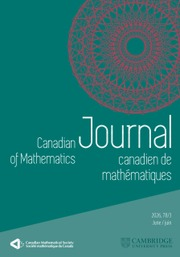Canadian Journal of Mathematics

# Non-stable K1-functors of Multiloop Groups

Published online by Cambridge University Press:  20 November 2018

## Abstract

HTML view is not available for this content. However, as you have access to this content, a full PDF is available via the ‘Save PDF’ action button.

Let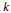$k$ be a field of characteristic 0. Let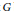$G$ be a reductive group over the ring of Laurent polynomials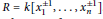$R\,=\,k\left[ x_{1}^{\pm 1},\ldots ,x_{n}^{\pm 1} \right]$ . Assume that$G$ contains a maximal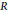$R$ -torus, and that every semisimple normal subgroup of$G$ contains a two-dimensional split torus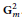$\mathbf{G}_{m}^{2}$ . We show that the natural map of non-stable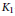${{K}_{1}}$ -functors, also called Whitehead groups,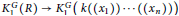$K_{1}^{G}\left( R \right)\,\to \,K_{1}^{G}\left( k\left( \left( {{x}_{1}} \right) \right)\cdots \left( \left( {{x}_{n}} \right) \right) \right)$ is injective, and an isomorphism if$G$ is semisimple. As an application, we provide a way to compute the difference between the full automorphism group of a Lie torus (in the sense of Yoshii–Neher) and the subgroup generated by exponential automorphisms.

## Keywords

Type
Research Article
Information
Canadian Journal of Mathematics , 01 February 2016 , pp. 150 - 178

## References

[A] Abe, E., Whitehead groups of Chevalley groups over polynomial rings. Comm. Algebra 11(1983), 12711307. http://dx.doi.org/10.1080/00927878308822906 CrossRefGoogle Scholar
[Ab] Abramenko, P., On finite and elementary generation of SL2(R). arxiv:0808.1095Google Scholar
[AABGP] Allison, B., Azam, S., Berman, S., Gao, Y., and Pianzola, A., Extended affine Lie algebras and their root systems. Mem. Amer. Math. Soc. 126(1997), no. 603.http://dx.doi.Org/10.1090/memo/0603 Google Scholar
[ABFP] Allison, B., Berman, S., Faulkner, J., and Pianzola, A., Multiloop realization of extended affine Lie algebras and Lie tori. Trans. Amer. Math. Soc. 361(2009), 48074842.http://dx.doi.org/10.1090/S0002-9947-09-04828-4 CrossRefGoogle Scholar
[BaMo] Bachmuth, S. and Mochizuki, H. Y., E2 ≠ SL2 for most Laurent polynomial rings. Amer. J.Math. 104(1982), no. 6, 11811189.http://dx.doi.org/10.2307/2374056 CrossRefGoogle Scholar
[B] Bass, H., K-theory and stable algebra. Inst. Hautes Études Sci. Publ. Math. 22(1964), 560.CrossRefGoogle Scholar
[BT1] Borel, A. and Tits, J., Groupes réductifs. Inst. Hautes Études Sci. Publ. Math. 27(1965), 55151.CrossRefGoogle Scholar
[BT2] Borel, A. and Tits, J., Homomorphismes “abstraits” de groupes algébriques simples. Ann. of Math. 97(1973), 499571.http://dx.doi.Org/10.2307/1970833 CrossRefGoogle Scholar
[Bou] Bourbaki, N., Groupes et algèbres de Lie. Chapitres 4–6. Hermann, Paris, 1968.Google Scholar
[ChGPl] Chernousov, V., Gille, P., and Pianzola, A., Torsors over the punctured affine Une. Amer. J. Math. 134(2012), no. 6, 15411583.http://dx.doi.org/10.1353/ajm.2012.0051 CrossRefGoogle Scholar
[ChGP2] Chernousov, V., Gille, P., and Pianzola, A., Conjugacy classes for loop reductive group schemes and Lie algebras. Bull. Math. Sci. 4(2014), 281324.http://dx.doi.org/10.1007/s13373-014-0052-8 CrossRefGoogle Scholar
[ChGP3] Chernousov, V., Gille, P., and Pianzola, A., Whitehead groups of loop group schemes of nullity one. J. Ramanujan Math. Soc. 29(2014), no. 1, 126.Google Scholar
[ChM] Chernousov, V. and Merkurjev, A. S., R-equivalence and special unitary groups. J. Algebra 209(1998), 175198.http://dx.doi.Org/10.1006/jabr.1998.7534 CrossRefGoogle Scholar
[Che] Chevalley, C., Certains schémas de groupes semi-simples. Sera. Bourbaki 6(1995), 219234.Google Scholar
[SGA3] Demazure, M. and Grothendieck, A. , Schémas en groupes. Lecture Notes in Math., 151–153, Springer-Verlag, Berlin-Heidelberg-New York, 1970.Google Scholar
[FGIKNV] Fantechi, B., Göttsche, L., Illusie, L., Kleiman, S., Nitsure, N., and Vistoli, A., Fundamental algebraic geometry. Grothendieck's FGA explained. Mathematical Surveys and Monographs, 123, American Mathematical Society, Providence, RI, 2005.Google Scholar
[Gl] Gille, P., Spécialisation de la R-équivalence pour les groupes réductifs. Trans. Amer. Math. Soc. 356(2004), 44654474.http://dx.doi.org/10.1090/S0002-9947-04-03443-9 CrossRefGoogle Scholar
[G2] Gille, P., Le problème de Kneser-Tits. Séminaire Bourbaki Vo. 2007/2008, Astérisque 326(2009), Exp. No. 983, vii, 3981.Google Scholar
[GP1] Gille, P. and Pianzola, A., Galois cohomology and forms of algebras over Laurent polynomial rings. Math. Ann. 338(2007), 497543.http://dx.doi.Org/10.1007/s00208-007-0086-2 CrossRefGoogle Scholar
[GP2] Gille, P. and Pianzola, A., Isotriviality and étale cohomology of Laurent polynomial rings. J. Pure Appl. Algebra 212(2008), 780800.http://dx.doi.Org/10.1016/j.jpaa.2007.07.005 CrossRefGoogle Scholar
[GP3] Gille, P. and Pianzola, A., Torsors, reductive group schemes and extended affine Lie algebras. Mem. Amer. Math. Soc. 226(2013), no. 1063.Google Scholar
[HV] Hazrat, R. and Vavilov, N., K1 of Chevalley groups are nilpotent. J. Pure Appl. Algebra 179(2003), 99116.http://dx.doi.org/10.1016/S0022-4049(02)00292-X CrossRefGoogle Scholar
[J] Jardine, J. F., On the homotopy groups of algebraic groups. J. Algebra 81(1983), 180201. http://dx.doi.Org/10.1016/0021-8693(83)90215-6 CrossRefGoogle Scholar
[Ma] Manin, Yu. I., Cubic forms: algebra, geometry, arithmetic. 2nd ed., North Holland Mathematical Library, 4, North Holland, Amsterdam, 1986.Google Scholar
[M] Margaux, B., The structure of the group G(k[t]): Variations on a theme of Soulé. Algebra Number Theory 3(2009), 393409.http://dx.doi.org/10.2140/ant.2009.3393 CrossRefGoogle Scholar
[N] Neher, E., Lie tori. C. R. Math. Acad. Sci. Soc. R. Can. 26(2004), no. 3, 8489.Google Scholar
[OPa] Ojanguren, M. and Panin, I., Rationally trivial hermitian spaces are locally trivial. Math. Z. 237(2001), 181198.http://dx.doi.org/10.1007/PL00004859 CrossRefGoogle Scholar
[PaStV] Panin, I., Stavrova, A., and Vavilov, N., On Grothendieck-Serre's conjecture concerning principal G-bundles over reductive group schemes: I. Compos. Math. 151(2015), no. 3,535567.http://dx.doi.Org/10.1112/S0010437X14007635 CrossRefGoogle Scholar
[PStl] Petrov, V. and Stavrova, A., Elementary subgroups of isotropic reductive groups. St. Petersburg Math. J. 20(2009), no. 4, 625644.http://dx.doi.Org/10.1090/S1061-0022-09-01064-4 CrossRefGoogle Scholar
[PSt2] Petrov, V. and Stavrova, A., Tits indices over semilocal rings. Transform. Groups 16(2011), 193.–217 http://dx.doi.Org/10.1007/s00031-010-9112-7 CrossRefGoogle Scholar
[Q] Quillen, D., Projective modules over polynomial rings. Invent. Math. 36(1976), 167171.http://dx.doi.org/10.1007/BF01390008 CrossRefGoogle Scholar
[Se] Serre, J.-P., Galois cohomology. English transi, by P. Ion, Springer-Verlag, Berlin Heidelberg, 1997.Google Scholar
[StO9] Stavrova, A., Stroenije isotropnyh reduktivnyh grupp. Ph.D. dissertation, St. Petersburg State University, 2009.Google Scholar
[Stl3] Stavrova, A., Homotopy invariance of non-stable K1-functors. J. K-Theory 13(2014), 199248. http://dx.doi.Org/10.1017/isOl3006012jkt232 CrossRefGoogle Scholar
[S78] Stein, M. R., Stability theorems for K1, K2 and related functors modeled on Chevalley groups. Japan J. Math. (N.S.) 4(1978), no. 1, 77108.CrossRefGoogle Scholar
[Su] Suslin, A. A., On the structure of the special linear group over polynomial rings. Math. USSR Izv. 11(1977), 221238.CrossRefGoogle Scholar
[Tl] Tits, J., Algebraic and abstract simple groups. Ann. of Math. 80(1964), 313329.http://dx.doi.org/10.2307/1970394 CrossRefGoogle Scholar
[V] Voskresenskiĭ, V. E., Algebraic groups and their birational invariants. Translations of Mathematical Monographs, 179, American Mathematical Society, Providence, RI, 1998.Google Scholar
[W] Wendt, M.,-homotopy of Chevalley groups. J. K-Theory 5(2010), 245287.http://dx.doi.Org/10.1017/isO10001014jktO96 CrossRefGoogle Scholar
[Y] Yoshii, Y., Lie tori-A simple characterization of extended affine Lie algebras. Publ. Res. Inst. Math. Sci. 42(2006), 739762. http://dx.doi.org/10.2977/prims/11666421 58 CrossRefGoogle ScholarYou have Access
3
Cited by

# Send article to Kindle

Note you can select to send to either the @free.kindle.com or @kindle.com variations. ‘@free.kindle.com’ emails are free but can only be sent to your device when it is connected to wi-fi. ‘@kindle.com’ emails can be delivered even when you are not connected to wi-fi, but note that service fees apply.

Find out more about the Kindle Personal Document Service.

Non-stable K1-functors of Multiloop Groups
Available formats
×

# Send article to Dropbox

To send this article to your Dropbox account, please select one or more formats and confirm that you agree to abide by our usage policies. If this is the first time you use this feature, you will be asked to authorise Cambridge Core to connect with your <service> account. Find out more about sending content to Dropbox.

Non-stable K1-functors of Multiloop Groups
Available formats
×

# Send article to Google Drive

To send this article to your Google Drive account, please select one or more formats and confirm that you agree to abide by our usage policies. If this is the first time you use this feature, you will be asked to authorise Cambridge Core to connect with your <service> account. Find out more about sending content to Google Drive.

Non-stable K1-functors of Multiloop Groups
Available formats
×
×